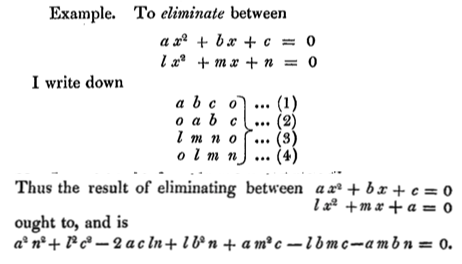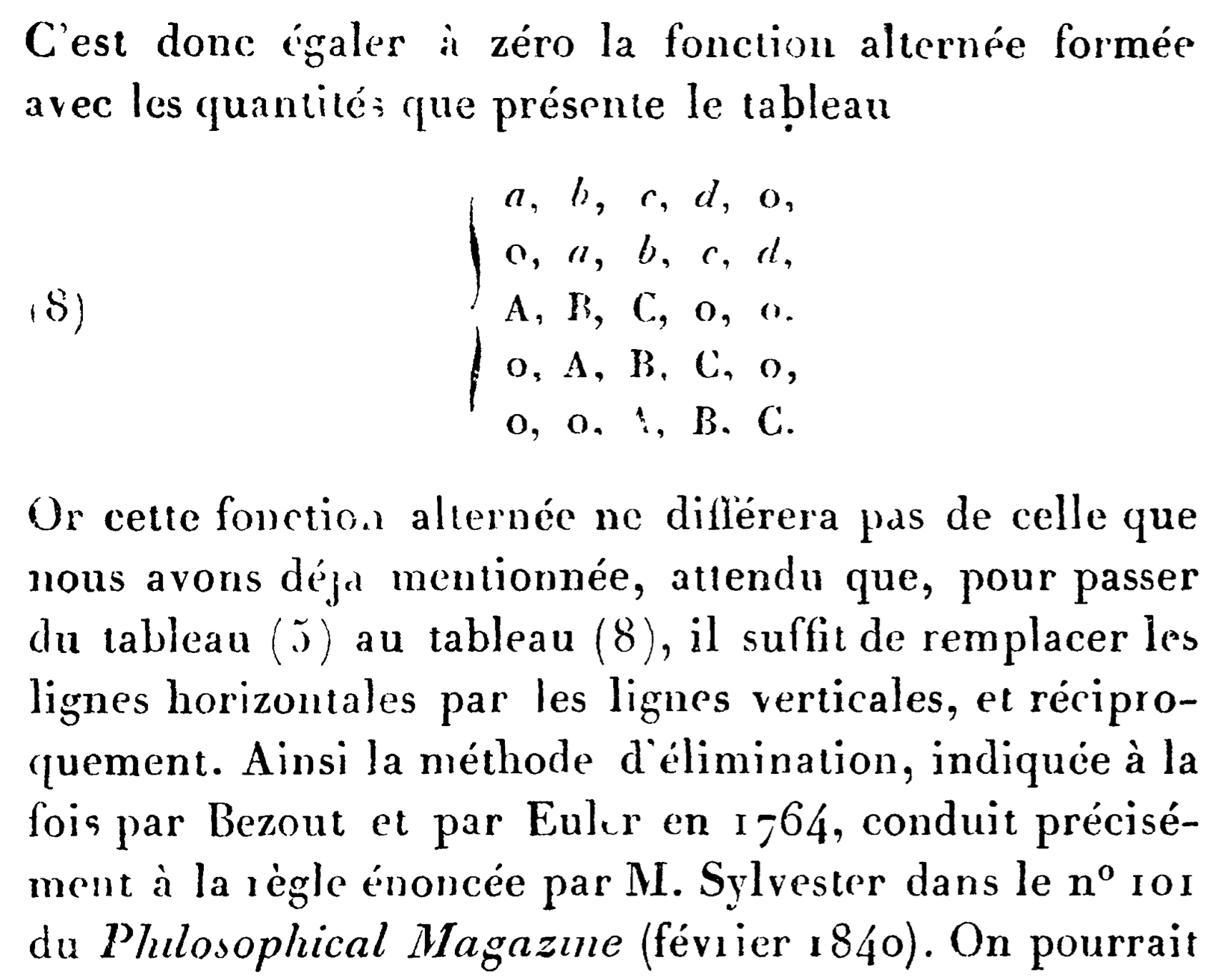# History of Sylvester's resultant?

Suppose that we have two polynomials that split: \begin{align*} f(x)=\sum_{k=0}^d a_{d-k}x^k&=\prod_{i=1}^d (x-\lambda_i),\\ g(x)=\sum_{k=0}^e b_{e-k}x^k&=\prod_{j=1}^e (x-\mu_j).\\ \end{align*} Then the following result is often attributed to James Joseph Sylvester: $$\det\begin{pmatrix} a_0 & a_1 & \cdots & a_d && \\ & \ddots &\ddots &&\ddots & \\ && a_0 & a_1 & \cdots & a_d \\ b_0 & b_1 & \cdots & b_e && \\ & \ddots & \ddots && \ddots & \\ && b_0 & b_1 & \cdots & b_e \end{pmatrix} = \prod_{i,j} (\lambda_i-\mu_j).$$

Can someone help me track down the original reference?

• The history of resultants is quite complicated. I am not familiar with non European contributions, but early European work on this is by Stevin 1585, Leibnitz 1683. A good reference (in French) is the article from the encyclopedia of math see, starting from page 73, books.google.com/… Oct 29, 2020 at 14:58
• This question might be better on hsm.stackexchange.com Oct 29, 2020 at 14:59

The resultant of the Sylvester matrix first appeared in J. J. Sylvester, Philos. Magazine 16, 132–135 (1840): A method of determining by mere inspection the derivatives from two equations of any degree.The last line is the resultant of the two equations at the top, which vanishes when two roots coincide.
I like the "ought to, and is" phrasing...

The Sylvester matrix also appeared in an 1840 publication by Cauchy, who acknowledged the priority of Sylvester:1876 reprint of Cauchy's 1840 article "Mémoire sur l'élimination d'une variable entre deux équations algébriques".

The name "resultant" originates from

E. Bézout (1764): Recherches sur le degré des équations résultantes de l'évanouissement des inconnues et sur les moyens qu'on doit employer pour trouver ces équations.
Research on the degree of equations resulting from the vanishing of unknowns and on the methods one should use to obtain these equations.

• It's always amazing to look back a few centuries and see how styles of exposition, and even of paper names, change over time. Oct 29, 2020 at 19:19
• Section 9 of this article has a nice discussion of the history. Oct 29, 2020 at 22:57
• @DrewArmstrong's reference: Penchèvre - Etienne Bézout on elimination theory. Nov 1, 2020 at 14:33